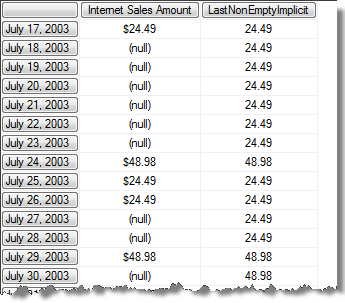MDX: Implicit Recursion

A few months ago Richard Lees did a blog post showing how you can calculate a LastNonEmpty measure using recursion. In Richard's example he used what I call "explicit recursion" in that he explicitly referenced the measure in it's own expression.

An example of this sort of calculation against Adventure Works would look like the following.

WITH
MEMBER Measures.LastNonEmptyExplicit
AS IIF(IsEmpty(Measures.[Internet Sales Amount])
,([Date].[Calendar].PrevMember, Measures.[LastNonEmptyExplicit])
,Measures.[Internet Sales Amount])
SELECT
{[Measures].[Internet Sales Amount]
,[Measures].[LastNonEmptyExplicit] } ON COLUMNS,
TAIL([Date].[Calendar].[Month].&&.Children,15)
ON ROWS
WHERE [Product].[Product Categories].[Subcategory].[Gloves]

If you run this you will see results like the following, I have included the raw measure so that you can see that way the calculation carries the last non-empty value forward. I have drawn in some arrows so you can see how the calculation would evaluate for the July 23 figure.However as the title of this post would suggest, there is another variation on the syntax for doing recursion which I call "implicit recursion" and it looks like the following

WITH
MEMBER Measures.LastNonEmptyImplicit
AS IIF(IsEmpty(Measures.[Internet Sales Amount])
,([Date].[Calendar].PrevMember)
,Measures.[Internet Sales Amount])
SELECT
{[Measures].[Internet Sales Amount]
,[Measures].[LastNonEmptyImplicit] } ON COLUMNS,
TAIL([Date].[Calendar].[Month].&&.Children,15)
ON ROWS
WHERE [Product].[Product Categories].[Subcategory].[Gloves]

Note that the output is identical.The only difference between these two examples is that the second does not explicitly reference itself, but because there is a .PrevMember call on the calendar date hierarchy and no other measure is reference the use of the current measure is implied. This is very subtle and I have seen it done accidentally before which lead to a lot of confusion.

Effectively "implicit recursion" is evaluated the same as if you had entered the following.

WITH
MEMBER Measures.LastNonEmptyImplicit
AS IIF(IsEmpty(Measures.[Internet Sales Amount])
,([Date].[Calendar].PrevMember, Measures.CurrentMember)
,Measures.[Internet Sales Amount])

And because at the point where the expression is evaluated the "CurrentMember" on the measures dimension is the calculation itself we end up with a recursion.

So my advice is to always use the "explicit" version when doing recursion. If you have a recursive measure in your MDX Script I would even go so far as to suggest putting a comment indicating that it is a recursive measure. Recursive measures are really powerful and are an extremely elegant coding technique, but they do have a performance impact, so you want to make sure that you are using them explicitly and that they are clearly identified.

Print | posted on Saturday, November 6, 2010 6:21 AM